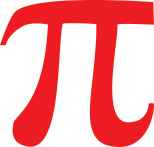Pi Day

The number Pi = 3.14159 has special significance in mathematics and any birthday date can be found somewhere in this number. Following the American tradition, Pi Day takes place annually on March 14th (3/14). Students are invited to work on mathematical problems related to the number Pi.

For over 10 years at GISW, grades 5 and 6, 7 and 8, 9 and 10, 11 and 12 have competed as teams to solve mathematical problems and illustrate their ways for solutions. A total of 270 students are active and engaged with mathematics on this day. The results of the best teams are evaluated by committed teachers of the math department and the winner of each level ofcompetition then traditionally receives an edible pie.

< back